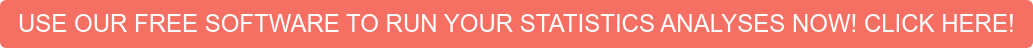# Statistical Power Analysis

Power analysis is directly related to tests of hypotheses. While conducting tests of hypotheses, the researcher can commit two types of errors: Type I error and Type II error. Statistical power mainly deals with Type II errors.

It should be noted by the researcher that the larger the size of the sample, the easier it is for the researcher to achieve the 0.05 level of significance.   If the sample is too small, however, then the investigator might commit a Type II error due to insufficient power.

Power analysis is normally conducted before the data collection.  The main purpose underlying power analysis is to help the researcher to determine the smallest sample size that is suitable to detect the effect of a given test at the desired level of significance.  The reason for applying power analysis is that, ideally, the investigator desires a smaller sample because larger samples are often costlier than smaller samples.  Smaller samples also optimize the significance testing.Factors That Affect Power

There are certain factors that affect power in power analysis:

The desired power level affects the power in analysis to a great extent.  The desired power level is typically 0.80, but the researcher performing power analysis can specify the higher level, such as 0.90, which means that there is a 90% probability the researcher will not commit a type II error.

One of the stringent factors in power analysis is the desired level of significance.  Suppose the researcher specifies 0.001 as the level of significance.  In this case, the power in power analysis will be decreased.  Thus, an alpha level of 0.001 is applicable only in those situations in which the researcher is mainly interested in avoiding a Type I error.

Another factor affecting the power of an analysis is the strength of association or the strength of relationship between the two variables.  The greater this strength of association is, the more the power in the power analysis.  This means that a greater strength of association leads to a greater value of power in power analysis.

A factor called sensitivity affects the power in power analysis.  The term sensitivity refers to the number of true positives out of the total of true positives and false negatives. In other words, this effect of power analysis recognizes the truly corrected data.  This means that highly sensitive data will yield data with higher value of power in power analysis, which means that the researcher will be less likely to commit Type II error from this data.

The variation of the dependent variable also affects the power.  The larger the variation in the dependent variable is, the greater the likelihood of committing Type II errors by the researcher. This means that the value of the power will be lower in power analysis.Assumptions of Power Analysis

There are two assumptions in an analysis of power.  The first assumption of analysis involves random sampling.  This means that the sample on which power analysis is being conducted is drawn by the process of random sampling.

Limitations

There are also certain limitations of the analysis of power.  The researchers should know the factors that affect the power are not taken into account by certain software packages.  Power analysis by certain software may recommend lower sample sizes than the ideal sample size for a given procedure.  In other words, power analysis generates certain guidelines for the size of the sample but cannot reflect the complexities that a researcher comes across while doing certain research projects.

Statistics Solutions can assist with determining the sample size / power analysis for your research study. To learn more, visit our webpage on sample size / power analysis, or contact us today.

Additional Resource Pages Related to Statistical Power Analysis: